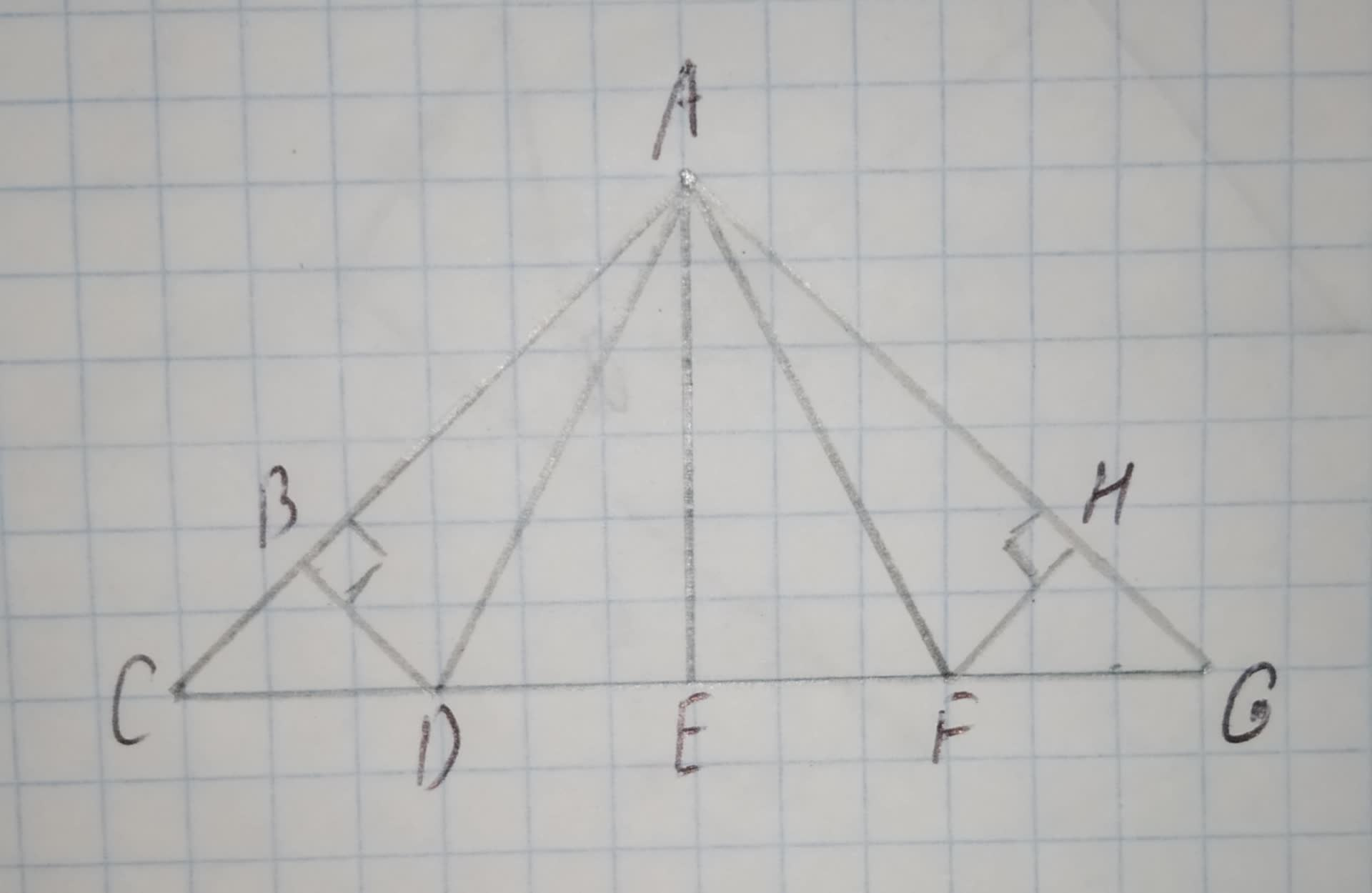# In the roof truss shown, AB = 8 and m/_HAF = 37^(circ). Find: m/_ADB. (HINT: The design of the roof truss displays line symmetry.) 12210203661.jpgvestirme4 2020-11-05 Answered
In the roof truss shown, AB = 8 and $m\mathrm{\angle }HAF={37}^{\circ }$. Find: $m\mathrm{\angle }ADB$.
(HINT: The design of the roof truss displays line symmetry.)You can still ask an expert for help

## Want to know more about Congruence?

• Questions are typically answered in as fast as 30 minutes

Solve your problem for the price of one coffee

• Math expert for every subject
• Pay only if we can solve itsaiyansruleA
Find the value of $m\mathrm{\angle }ADB$.
The sum of measure of three angles of the triangle is ${180}^{\circ }$
$m\mathrm{\angle }ADB+m\mathrm{\angle }BAD+m\mathrm{\angle }ABD={180}^{\circ }$
${37}^{\circ }+{90}^{\circ }+m\mathrm{\angle }ADB={180}^{\circ }$
$m\mathrm{\angle }ADB={180}^{\circ }-{37}^{\circ }-{90}^{\circ }$
$m\angle ADB={53}^{\circ }$
Hence, the value of $m\mathrm{\angle }ADBis{53}^{\circ }$